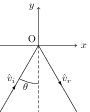# Reflection and Refraction at Plane and Spherical Surfaces

## Problems from IIT JEE

Problem (IIT JEE 2013): A ray of light travelling in the direction $\frac{1}{2}\left(\hat{\imath}+\sqrt{3}\,\hat{\jmath}\right)$ is incident on a plane mirror. After reflection, it travels along $\frac{1}{2}\left(\hat{\imath}-\sqrt{3}\,\hat{\jmath}\right)$. The angle of incidence is,

1. 30 degree
2. 45 degree
3. 60 degree
4. 75 degree

Solution:Let $\hat{v}_i=\frac{1}{2}\left(\imath+\sqrt{3}\jmath\right)$ and $\hat{v}_r=\frac{1}{2}\left(\imath-\sqrt{3}\jmath\right)$ be the unit vectors along the incident and the reflected rays. From Snell's law, angle of incidence (say $\theta$) is equal to the angle of reflection. Thus, angle between $-\hat{v}_i$ and $\hat{v}_r$ is $2\theta$ (see figure). Using, dot products of vectors, we get, \begin{align} \cos(2\theta)=- \hat{v}_i\cdot \hat{v}_r={1}/{2}. \nonumber \end{align} Hence, $\theta={30}\;\mathrm{degree}$. The readers can see that $\hat{v}_i=\cos 60\,\hat{\imath}+\sin 60\,\hat{\jmath}$ and $\hat{v}_r=\cos (-60)\,\hat{\imath}+\sin (-60)\,\hat{\jmath}$. Drawing these vectors gives desired result.